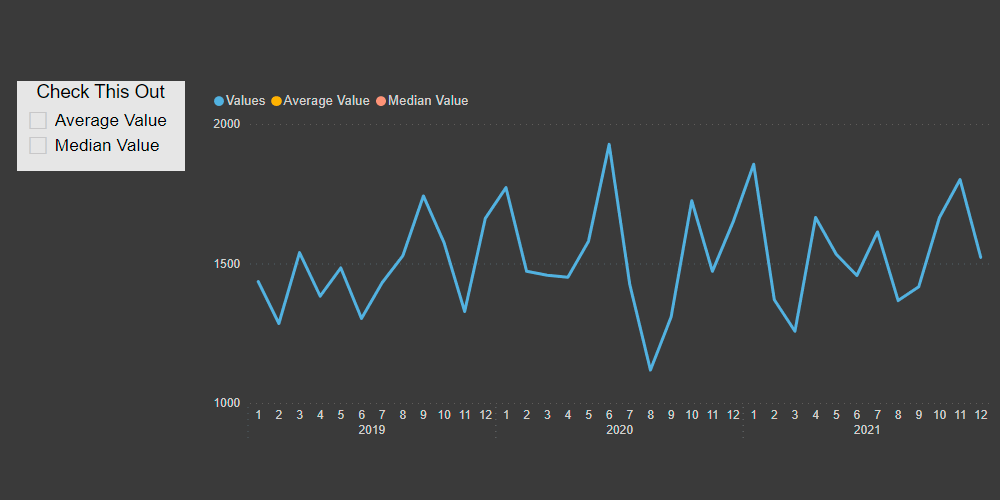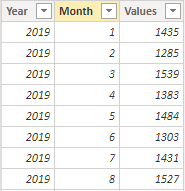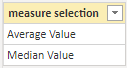# Control Power BI DAX measures with a slicer

It is easy to control Power BI DAX measures with a slicer and turn them on or off for visualizations or other purposes. Here is how to do that in 4 easy steps.

For demonstration purposes, I generated the data set in Power Query called “my data” the same way as in the previous post. You can check that out and copy M code to the blank query to recreate these examples.Let’s say I want to create a slicer that can add to my line chart additional series. For example, average and median, but you can also create more complex DAX measures – moving average, moving sum, outlier detection, data from the same week last year, etc.

## Here is how to create a Power BI DAX measure slicer in 4 easy steps

1. Create a table that contains a column with names that represent measures. In my case table is called “measure selection” with the same column name.2. Create a measure that checks whether a specific choice is filtered. That is done with the function ISFILTERED and SEARCH. ISFILTERED look for a fact of filter usage. SEARCH for a measure name in a string of all selected slicer items. If all that is true, then return the desired measure.

For the average value, it looks like this.

```Average Value =
VAR filtered =
ISFILTERED ( 'measure selection'[measure selection] )
&& SEARCH (
"Average",
CONCATENATEX (
ALLSELECTED ( 'measure selection'[measure selection] ),
'measure selection'[measure selection]
),
,
BLANK ()
)
VAR avrg =
CALCULATE ( AVERAGE ( 'my data'[Values] ), ALL ( 'my data' ) )
RETURN
IF ( filtered, avrg )
```

As you can see with the last argument in the SEARCH function, I’m making sure that there is no error if something is not found. That is one of the differences between DAX and similar Excel functions.

3. Add the column from the table where the names are the same as DAX measures to the slicer. In my case, column “measure selection” from table “measure selection”.

4. Add related measures to the line chart. Now you can turn them on or off and see desired information.That is how you can control Power BI DAX measures with a slicer depending on what is needed.

M code for the “my data” table.

```let
Source = List.Dates(#date(2019, 1, 1), 365 * 3, #duration(1, 0, 0, 0)),
#"Converted to Table" = Table.FromList(Source, Splitter.SplitByNothing(), null, null, ExtraValues.Error),
#"Renamed Columns" = Table.RenameColumns(#"Converted to Table",{{"Column1", "Date"}}),
#"Changed Type" = Table.TransformColumnTypes(#"Renamed Columns",{{"Date", type date}}),
#"Inserted Year" = Table.AddColumn(#"Changed Type", "Year", each Date.Year([Date]), Int64.Type),
#"Inserted Month" = Table.AddColumn(#"Inserted Year", "Month", each Date.Month([Date]), Int64.Type),